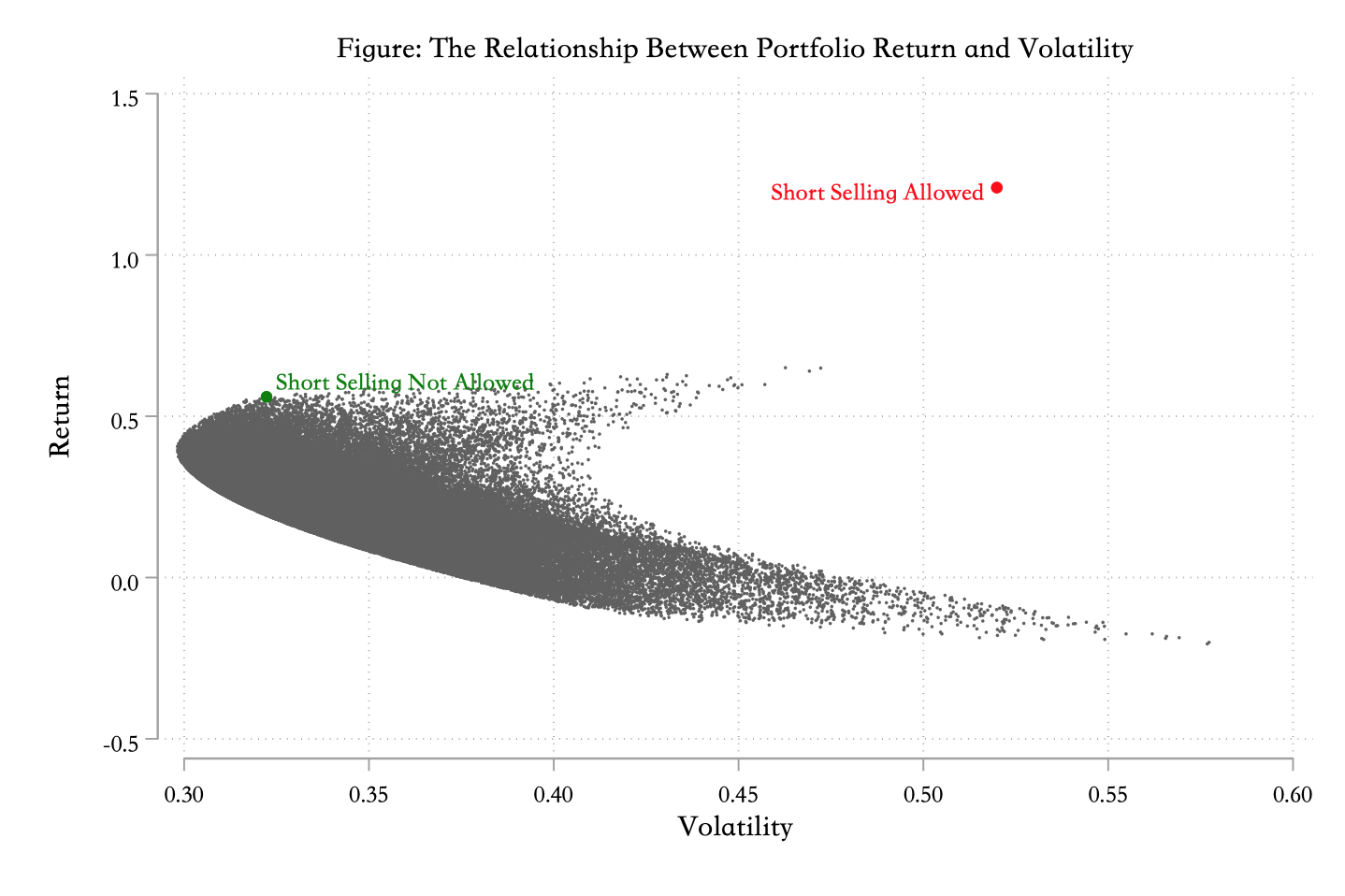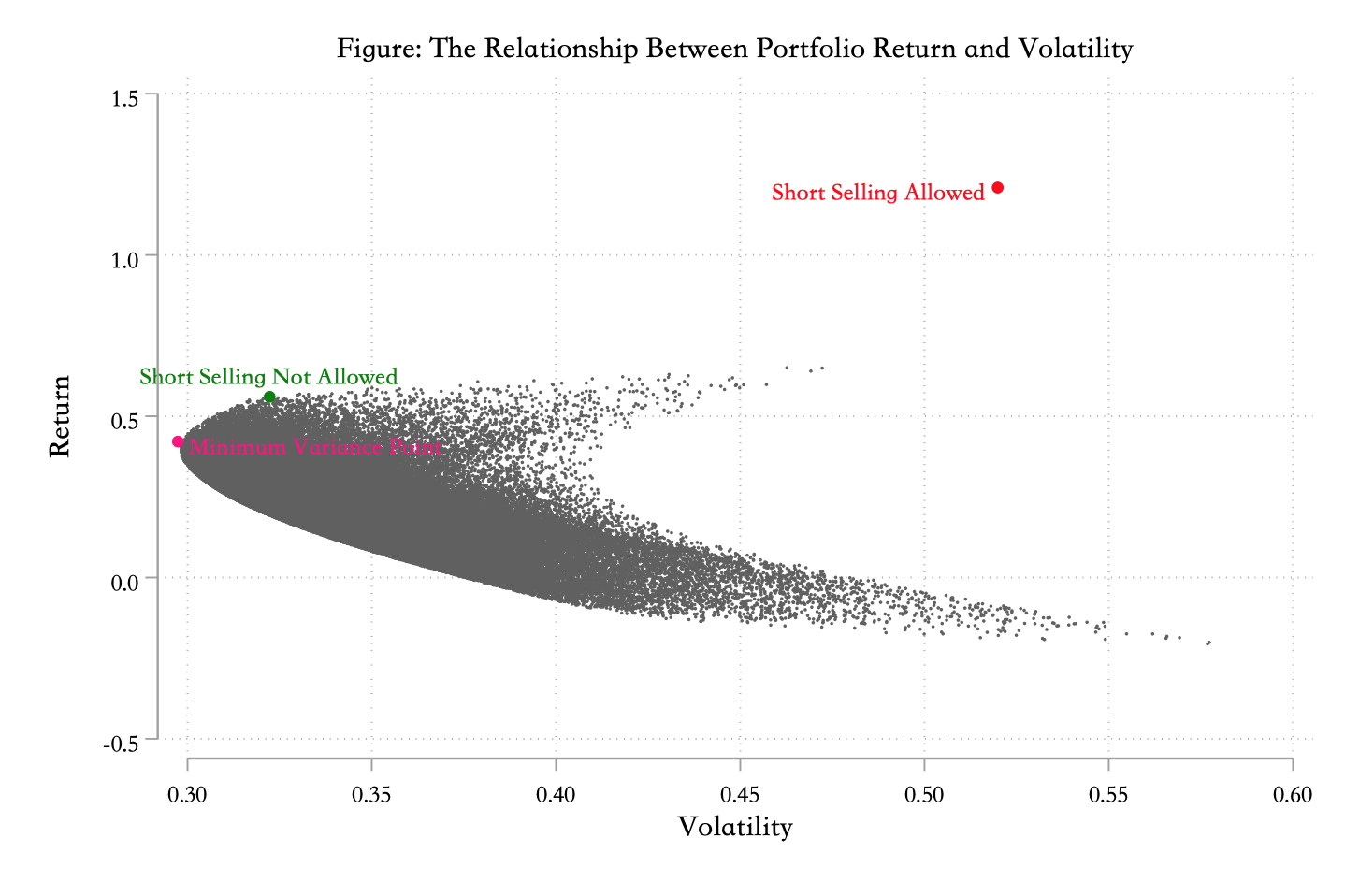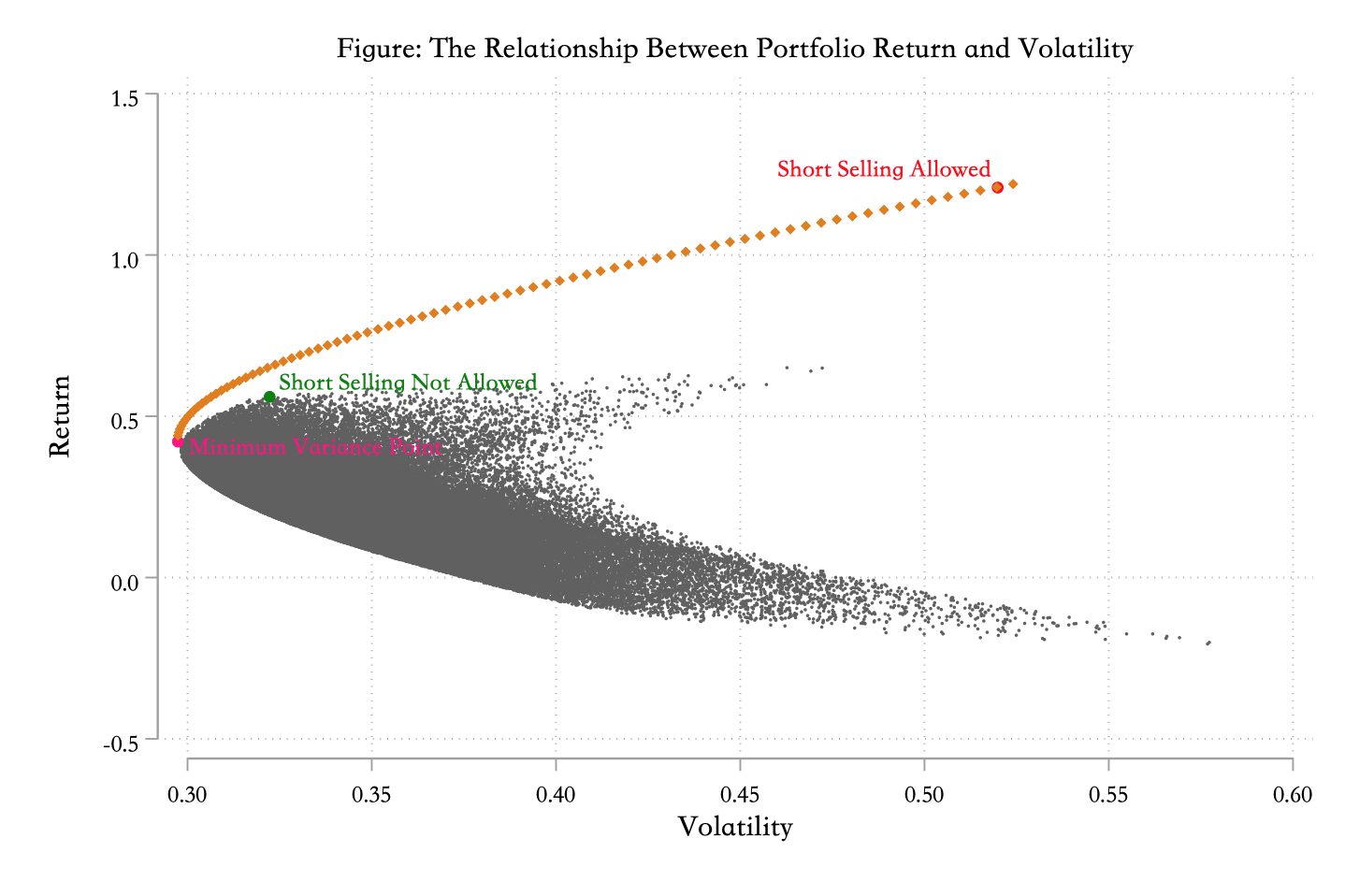# Stata Optimization and Markowitz Efficient Frontier

In my two previous tweets, I used Stata and Python to fit Markowitz Efficient Frontier respectively: Use Stata to Fine the Markowitz Efficient Frontier & Use Stata to Fine the Markowitz Efficient Frontier. But Stata verison is imperfection, because I don’t know how to optimize in Stata. This problem was finally solved today: How to optimize in Stata.

## Get stock data

First of all, review the previous code. This time, I used four stocks: Yintai Resource, Dayuecheng, China tianying, and Tonghua golden horse. The time range is from January 1, 2019 to today, August 14.

Here I use the cntrade2 command in my ‘finance’ package, about how to use this package, you can reference: FINANCE: Finance Toolkit in Stata. The cntrade2 command was mainly modified from ‘cntrade’ which written by ‘Crawler Club’.

Merge these four datesets horizontally:

## Observing the trend of stock price

Standardize the stock price at the begining of the period to 100, and observe the stock price trend of four stocks:Next, we calculate the logarithmic return of each stock. We need to pay attention to that there are intervals between trading days. So we can’t use directly use the ‘date’ variable as date index.

## Calculating the logarithmic returns

The formula of calculating the logarithmic returns:

$$R_{n} = \log{P_{n}} - \log{P_{n-1}}$$

where $P_{n}$ indicates stock price at $n$th day.

Observe the distribution of stock logarithmic returns:## Portfolio construction

### Maximizing Sharpe-ratio

Sharpe-ratio represents that how many excess returns can traders get when they take one unit additional risk. If it is positive, it means that the excess return rate is higher than the volatility. If it is negative, it means that the operational risk is higher than the excess return rate. In this way, each portfolio can calculate a Sharpe-ratio, that is, the ratio of return on investment to risk taking. The higher the Sharpe ratio, the better the corresponding portfolio.

$$Sharpe-ratio = \frac{R_{p} - R_{f}}{\sigma_{p}}$$

Where $R_{p}$ represents portfolio’s return. $R_{f}$ stand for risk-free interest rate which is assumed to be $4\%$, $\sigma_{p}$ is the standard deviation of portfolio returns, repersenting the volatility of portfolio returns.

Assuming that there are $n$ securities in the portfolio, the weight of security $i$ if $w_{i}$ with return rate at $r_{i}$. Then the standard deviation of the portfolio is: $\sqrt{w’Vw}$, where $w$ is the weight vector and $V$ is the variance-covariance matrix of the return rate vector. Next, we can write a program to maximize Sharpe-ratio. Now, we have four stocks, that is two say, we need to determine the weight of four stocks, Since the total weight should be 1, so we just need to determine three weight parameters.

First, calculate the logarithmic return of each stock and stored in a matrix named returns(actually a one-dimensional row vector).

Then, calculate the variance-covariance matrix, stored it in matrix variance:

The next step if to use Stata to optimize. Four your understanding, Let me give you an example from Stata help document. If I want to optimize $y = e^{-x^2 + x - 3}$:

mata: means to enter Mata language environment, then define a function named myeval, void means that the function has no return value. There are five parameters: $todo, x, y, g, H$. Here we just need to use $x$ and $y$.

That is to say, the parameters to be tuned are placed in the position of the second parameter, and the return value of the function is placed in the position of the third parameter.

S = optimize_init() indicates initialization, optimize_init_evaluator(S, &myeval()) set which function to be optimized, optimize_init_params(S, 0) set the initial parameter values， here is $x = 0$，The defaul optimization method is Newton–Raphson. At last, execute x = optimize(S) to optimize, $x$ is what we need.

First, we allow short selling which means the weight parameters can be negative.

Here $w123$ is a three-dimensional row vectol. The result of above codes is:

This it the portfolio with maximum Sharpe-ratio when we allow short selling.

Consider the short selling is not allowed. In order to ensure that the weight is positive and the sum is 1, I use the characteristics of the $e^x$ function.

And the result is:

### A Sharpe-ratio function

I also write a function to calculate the Sharpe-ratio:

The result is:

This is consistent with the results of above optimization.

When the short selling is allowed, the portfolio whit maximum Sharpe-ratio is to buy half of Yintai resource and half of Dayuecheng。

Next, we use Monte Carlo simulation to observe the relationship between the return and standard deviation of all possible combinations when short selling is allowed.

Display using graph:Obviously, short selling increases both returns and volatility.

### Minimizing variance

Minimizing variance equal to minimize standard deviation. which is the right-most point on the figure above. The following procedure is used to find the corresponding combination construction method for this point.

Note that in the code above, I put a negative sign in std = -sqrt(variancep[1, 1]) which minimizes the standard deviation when maximizing std.
The result is:

The location of this point is:## Constructing the efficient frontier

The efficient frontier is a line on which the point represents the portfolio with minimum volatility under given return rate. Obvious it is the boundary line from the minimum variance point to the maximum Sharpe-ratio point. From the above graph, we can see that the return rate range from the minimum variance to the maximum Sharpe-ratio point which allows short selling is [0.44, 1.77]. Then calculte each standard deviation of at the interval of 0.01.

Similarly, we plot these 134 points on the graph:Keytion